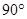### The Triangle and its Properties - Revision Notes

CBSE Class–VII Subject Mathematics
Revision Notes
Chapter – 6
The Triangle and its Properties

• Triangles: A closed plane figure bounded by three line segments.
• The six elements of a triangle are its three angles and the three sides.
• The line segment joining a vertex of a triangle to the mid point of its opposite side is called a median of the triangle. A triangle has 3 medians.
• The perpendicular line segment from a vertex of a triangle to its opposite side is called an altitude of the triangle. A triangle has 3 altitudes.
• Type of triangle based on Sides:
• Equilateral: A triangle is said to be equilateral, if each one of its sides has the same length. In an equilateral triangle, each angle has measure 60°.
• Isosceles: A triangle is said to be  isosceles, if atleast any two of its sides are of same length. The non-equal side of an isosceles triangle is called its base; the base angles of an isosceles triangle have equal measure.
• Scalene: A triangle having all side of different lengths. It has no two angles equal.
• Property of the lengths of sides of a triangle: The sum of the lengths of any two sides of a triangle is greater than the length of the third side.
• The difference between the lengths of any two sides is smaller than the length of the third side. This property is useful to know if it is possible to draw a triangle when the lengths of the three sides are known.
• Types of Triangle based on Angles:
(i) Right Angled Triangle: A triangle one of whose angles measures(ii) Obtused Angled Triangle: A triangle one of whose angles measures more than(iii) Acute Angled Triangle: A triangle each of whose angles measures less than• In a right angled triangle, the side opposite to the right angle is called the hypotenuse and the other two sides are called its legs.
• Pythagoras property: In a right-angled triangle, the square on the hypotenuse = the sum of the squares on its legs. If a triangle is not right-angled, this property does not hold good.  This property is useful to decide whether a given triangle is right-angled or not.
• An exterior angle of a triangle is formed, when a side of a triangle is produced. At each vertex, you have two ways of forming an exterior angle.
• A property of exterior angles: The measure of any exterior angle of a triangle is equal to the sum of the measures of its interior opposite angles.
• The angle sum property of a triangle: The total measure of the three angles of a triangle is 180°.
• Property of the Lengths of Sides of a Triangle: The sum of the lengths of any two sides of a triangle is always greater than the length of the third side. The difference of the lengths of any two sides of a triangle is always smaller than the length of the third side.